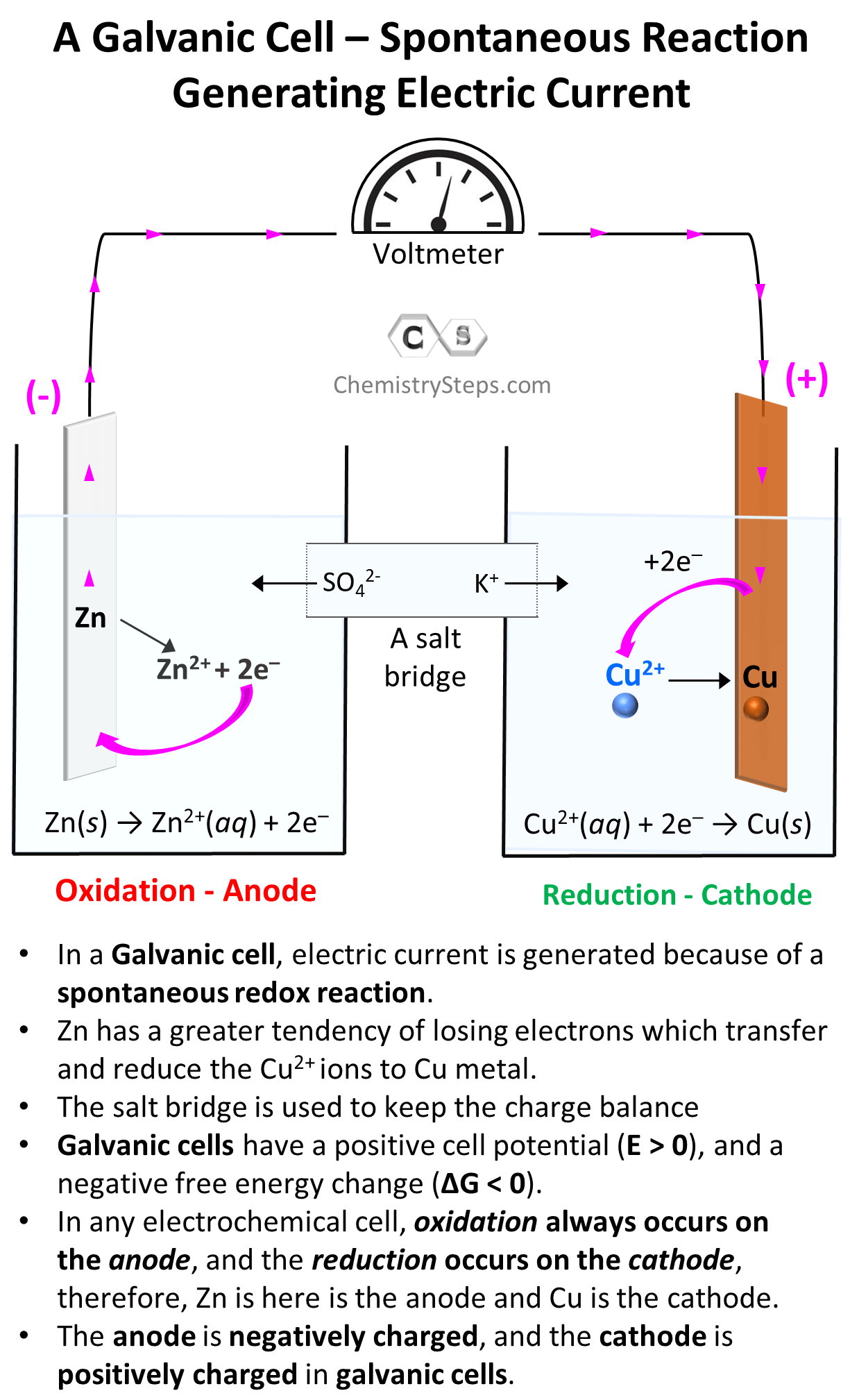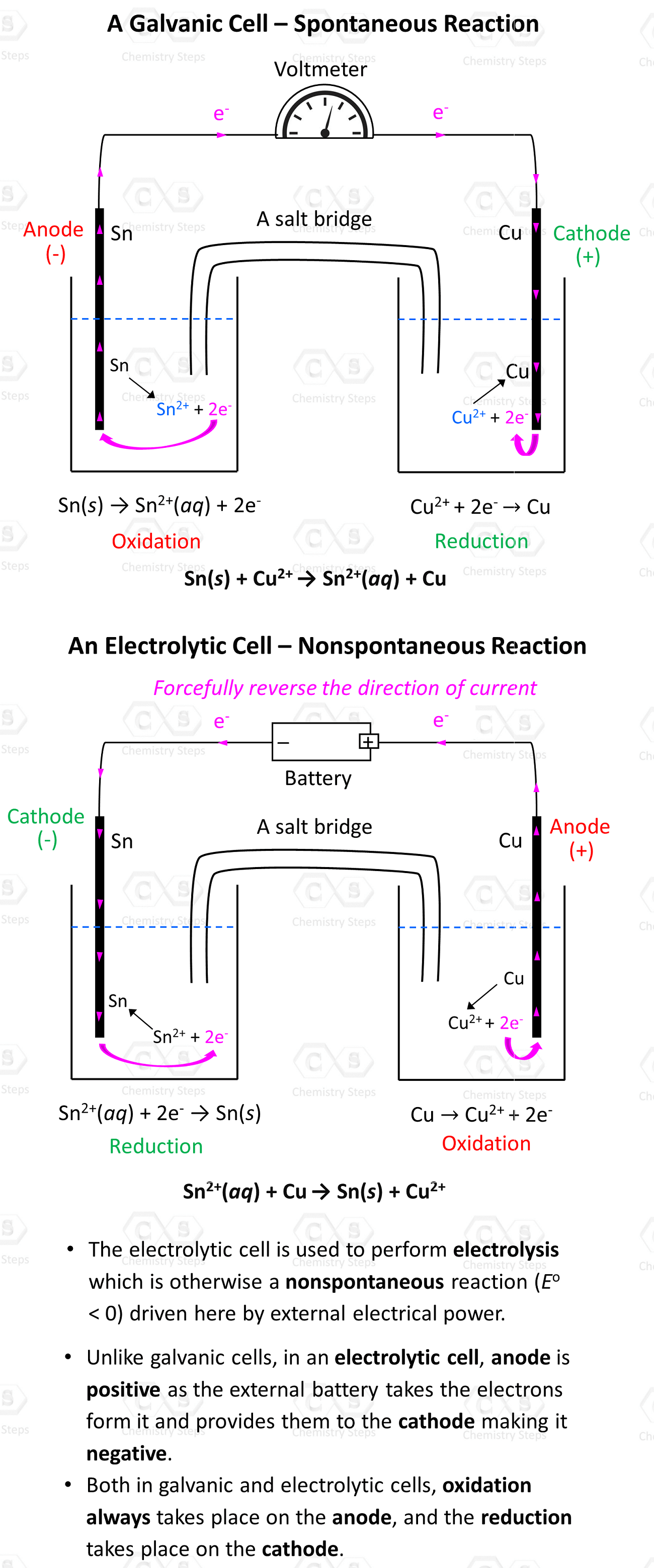## General Chemistry

Everything we discussed so far was about Galvanic cells which generate electric current because of spontaneous redox reactions. Galvanic cells, therefore, are characterized by positive cell potential (E) and negative free energyG).

The classic example for illustrating the principle of Galvanic cells is the reaction between Zn metal and Cu2+. Zn has a greater tendency for losing electrons and therefore, it gives the electrons to Cu2+ ions and thus reducing them to Cu. The key features of this reaction and Galvanic cells are summarized in the image below:Galvanic cells can be set up with any combination of metals and their electrolytes as long as there is an overall positive cell potential. For example, Cu2+ ions can also oxidize Sn to Sn2+ because of their higher reduction potential (greater tendency to be reduced) than Sn2+:

Sn(s) + Cu2+(aq) → Sn2+(aq) + Cu(s)

The values of half-reactions can be found in a standard textbook, and to find the cell potential, we simply add them after switching the sign for the reverse reaction.

Sn(s) → Sn2+(aq) + 2e–  E= +0.14 V

Cu2+(aq) + 2e–  → Cu(sE= +0.34 V
______________________________________

Sn(s) + Cu2+(aq) → Sn2+(aq) + Cu(sE= +0.48 V

Feel free to check this article for more details on calculating the standard cell potential.

Now, here is the question: what happens when the cell potential is negative? Will there be any reaction? The scenario for our example would be making Sn oxidize Cu to Cu2+:

Sn2+(aq) + Cu(s) → Sn(s) + Cu2+(aq) E= 0.48 V ?

Notice that the sign of Eo is switched to negative as we reverse the reaction and because of this the answer to the question above is no, there will be no reaction since the electrons won’t flow from Cu to Sn2+. But what if we supply some energy? Can we drive a nonspontaneous reaction with external electric power? And it turns out that yes, we can drive a nonspontaneous reaction and this process is called electrolysis which is carried out in Electrolytic cells. So, let’s see how an electrolytic cell is set up and how it is different from Galvanic cells.

# Electrolytic Cells

The first and main thing that is different in electrolytic cells is the use of an external power supply which can be, for example, a battery. Now, if we want to oxidize Cu, we are going to connect the positive end of the battery to the Cu electrode because it will pull the electrons and thus force oxidation:

Anode-oxidation: Cu(s) → Cu2+(aq) + 2e

The negative end of the battery is connected to species to be reduced (cathode), therefore we will connect to the Sn electrode to supply the electrons to Sn2+ ions.

Cathode-reduction: Sn2+(aq) + 2e → Sn(s)

Overall, here is how the Galvanic and Voltaic cells will differ for the two reactions:The external voltage must be higher than 0.48 V because that is the cell potential of the reverse reaction. With that said, it is not really Sn2+ that oxidizes Cu, but rather the external voltage. The metals and their electrolyte simply support the current flow by providing and accepting the electrons.

A few important differences of the electrolytic and galvanic cells:

• The electrolytic cell is used to perform electrolysis which is otherwise a nonspontaneous reaction (Eo< 0) driven here by external electrical power.
• Unlike galvanic cells, in an electrolytic cell, the anode is positive as the external battery takes the electrons from it and provides them to the cathode making it negative.
• Both in galvanic and electrolytic cells, oxidation always takes place on the anode, and the reduction takes place on the cathode.

In the next couple of articles, we will discuss electrolysis in more detail on the examples of molten and aqueous NaCl, as well the electrolysis of water.